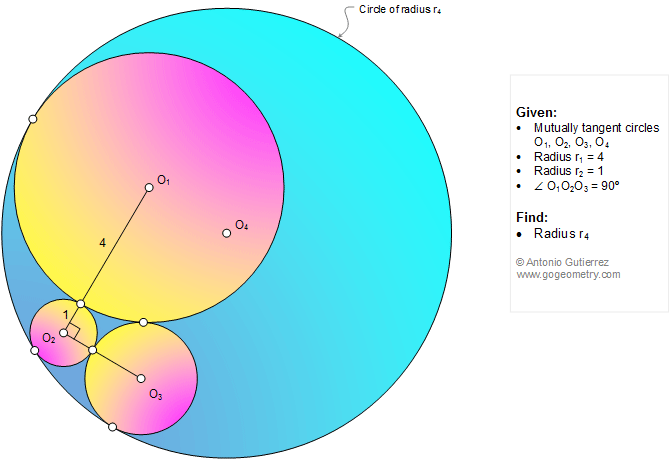# Geometry Problem 1157: Four Circles, Mutually Tangent, 90 Degrees, Radius, Metric Relations. Level: School, College, Mathematics Education

< PREVIOUS PROBLEM  |  NEXT PROBLEM >

 The figure below shows four mutually tangent circles O1, O2, O3, and O4 so that angle O1O2O3 = 90 degrees. If radius of circle O1 = 4 and radius of circle O2 = 1, find the radius of circle O4.Home | Search | Geometry | Problems | All Problems | Open Problems | Visual Index | 10 Problems | Problems Art Gallery | Art | 1151-1160 | Circle | Tangent Circles | Triangles | Right Triangle | 90 degrees | Metric Relations | Last updated: Oct 25 2015